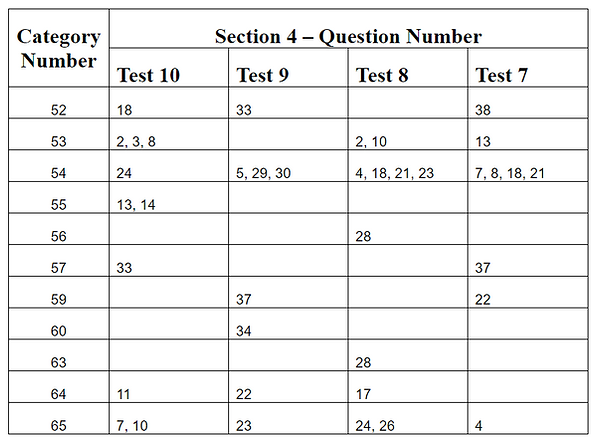• ## SAT Math question format

This book is written after a year of meticulous review and analysis of the math questions on the tests released by the College Board. The questions and the language of the questions in this book are based on these tests.

See few pages of examples, questions, and answers pages in this book by following THIS LINK.

• ## Concise and organized

This book is systematically organized as categories. Each category corresponds to a question-type on the SAT and starts with relevant concepts as bullet points (things that students normally highlight), followed by examples and practice questions.

• ## Clear and consistent

The examples and answers to practice questions are solved using one to three mathematical steps that will work on every question of a type, every single time. There are no tricks that only work on certain questions or may confuse a student rather than helping.

See how a student should be able to think and solve several questions without calculation or using mental math by following THIS LINK.

The book only contains the material that is necessary to answer questions on the SAT math sections and avoids overwhelming students with lengthy explanations. Students do not have to sift through paragraphs or pages or mark the book for material that is important.

• ## Complete and Balanced

All math areas outlined by the College Board for the SAT math sections are covered in this book. The book covers all the relevant topics with appropriate balance of material.

See how the categories in this book map to the solutions of SAT Practice Test 10 Sections 3 and 4 by following THIS LINK.

See below mapping of sections in this book to SAT topics outlined by the College Board and synopsis of Sections 3 and 11.

# Mapping of SAT Math Areas outlined by the College Board to the Sections in this BookSynopsis of Section 3 - Word Problems on Linear Equations and Inequalities

There are several word problems on linear equations and linear inequalities on the SAT Math Section 3 and Section 4. The approach to solve these word problems is relatively straightforward. They are an easy win for a student who is familiar with the types of word problems and the steps to solve them.

Section 3 of this book is devoted to word problems on linear equations and linear inequalities with one or two variables, and word problems on system of linear equations and linear inequalities. The categories in this section contain examples and practice questions on various types of word problems that appear on the SAT. There are total of 72 practice questions in this section.

Below table shows all the related questions that appeared on SAT Math Tests 7 through 10 and their mapping to categories in Section 3 of this book.Synopsis of Section 11 - Data Analysis and Interpretation

Every SAT Math Section 4 has several questions on topics related to probability, reading and interpreting graphs and events, statistical measures, and interpretation of data in studies and surveys. Section 11 of this book covers all the concepts that are required to answer the questions on the above mentioned topics. There are total of 131 practice questions in this section.

There may be questions on the SAT Math Section 4 that require analyzing multiple statistical measures in one data set or comparing multiple statistical measures in two data sets. This section contains a unique category, with examples and practice questions, that describes how to approach such questions.

Below table shows all the related questions that appeared on SAT Math Tests 7 through 10 and their mapping to categories in Section 11 of this book.